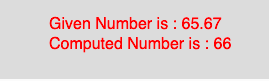# p5.js ceil() function

The ceil() function in p5.js is used to calculate the ceil value of a number. This function maps to the Math.ceil() of javascript. A number’s absolute value is always positive.

Syntax

```ceil(number)
```

Parameters: The function accepts only one parameter as mentioned above and described below:

• number : This parameter stores the number to compute.
• Below program illustrates the ceil() function in p5.js:
Example:

 `function` `setup() { ` `    ``//create Canvas of size 270*80   ` `    ``createCanvas(270, 80); ` `} ` ` `  `function` `draw() { ` `    ``background(220); ` `    ``//initialize the parameter   ` `    ``let x = 65.67; ` `    ``//call to ceil() function   ` `    ``let y = ceil(x); ` `    ``textSize(16); ` `    ``fill(color(``'red'``)); ` `    ``text(``"Given Number is : "` `+ x, 50, 30); ` `    ``text(``"Computed Number is : "` `+ y, 50, 50); ` `} `

Output:Reference: https://p5js.org/reference/#/p5/ceil

Whether you're preparing for your first job interview or aiming to upskill in this ever-evolving tech landscape, GeeksforGeeks Courses are your key to success. We provide top-quality content at affordable prices, all geared towards accelerating your growth in a time-bound manner. Join the millions we've already empowered, and we're here to do the same for you. Don't miss out - check it out now!

Previous
Next